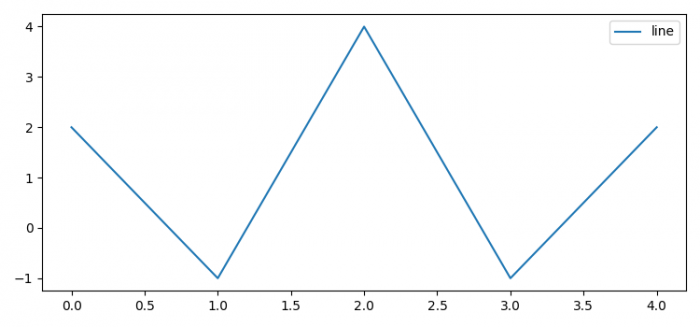# How to set label for an already plotted line in Matplotlib?

To set label for an already plotted line in Matplotlib, we can take the following steps −

• Set the figure size and adjust the padding between and around the subplots.
• Plot the line with an input list.
• Set the label of the created line.
• Place a legend on the plot at the "upper right" location.
• To display the figure, use show() method.

## Example

from matplotlib import pyplot as plt

plt.rcParams["figure.figsize"] = [7.50, 3.50]
plt.rcParams["figure.autolayout"] = True

line, = plt.plot([2, -1, 4, -1, 2])
line.set_label("line")

plt.legend(loc="upper right")

plt.show()

## Output